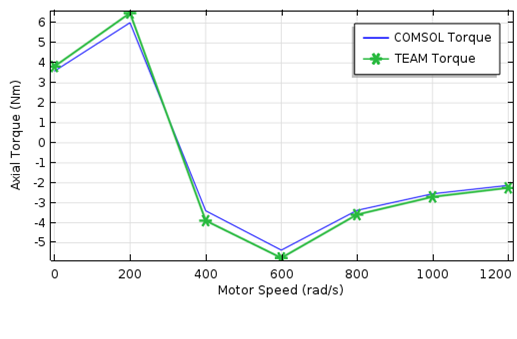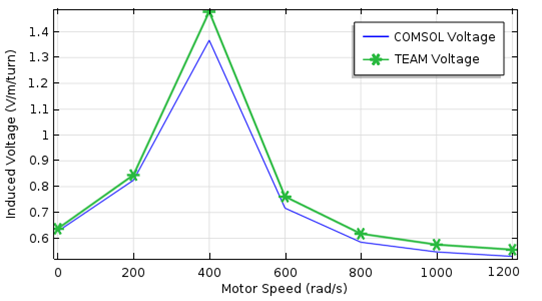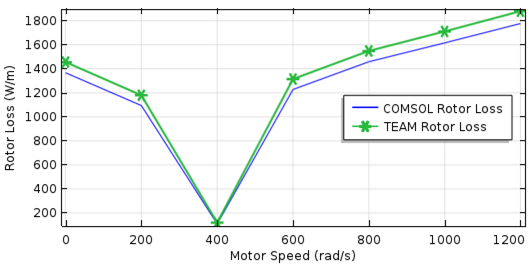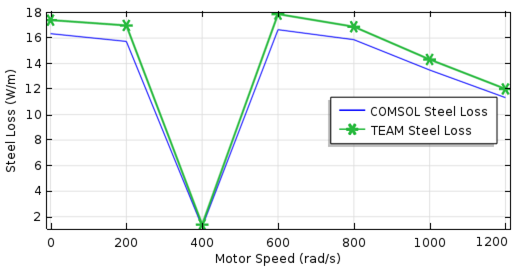# 如何分析感应电机：以测试电磁分析方法（TEAM）的标准模型为例

2016年 7月 18日

### 借助仿真创建感应电机模型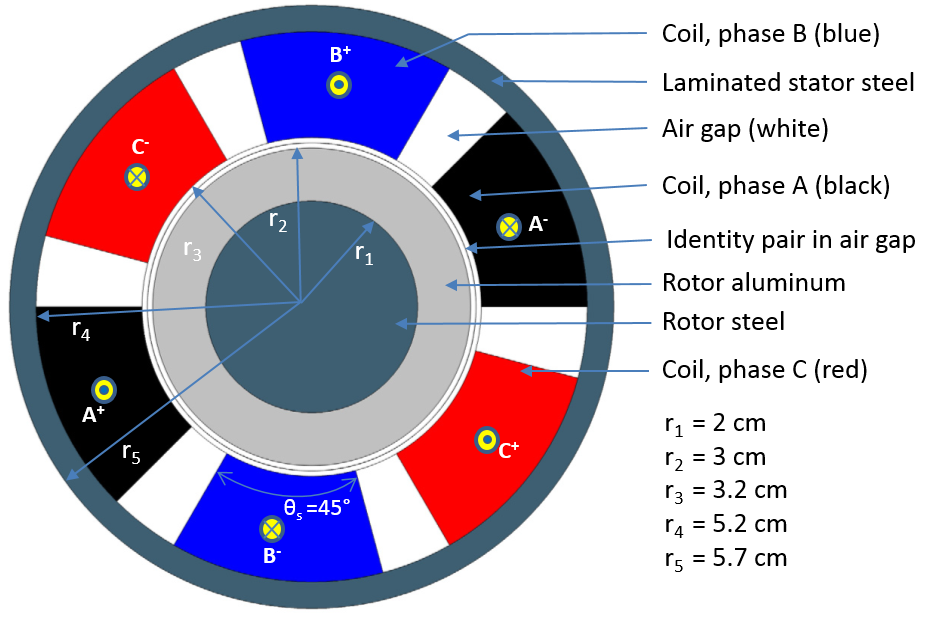### 在 COMSOL Multiphysics 中模拟感应电机的动力学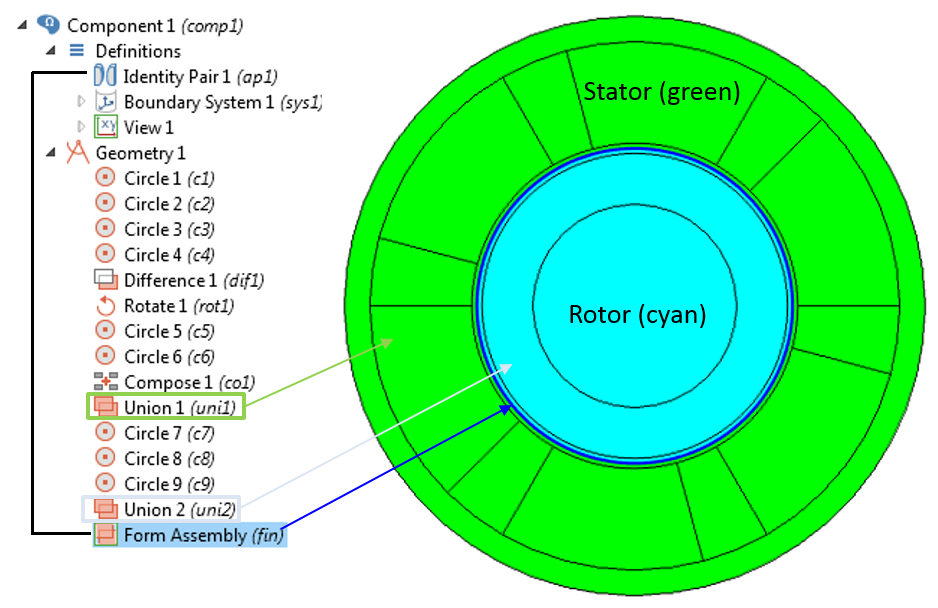1. I A = 1[A]*cos(w0*t)*sqrt(2)
2. I B = 1[A]*cos(w0*t+120[deg])*sqrt(2)
3. I C = 1[A]*cos(w0*t-120[deg])*sqrt(2)

T_e = \frac{1}{\mu_0(r_o-r_i)}\int\limits_{S_{ag}}rB_rB_\phi dS#### 使用全局常微分和微分代数方程接口模拟电机的动力学

(1)

\frac{d \omega_m}{dt}=\frac{T_m-T_L}{I}

(2)

\frac{d \phi}{dt}=\omega_m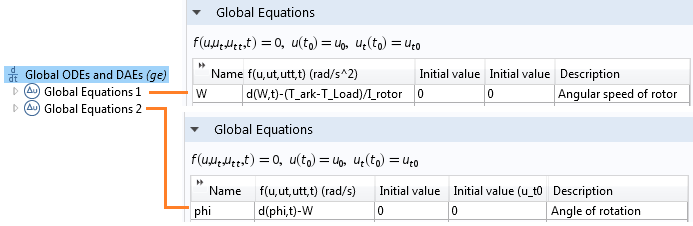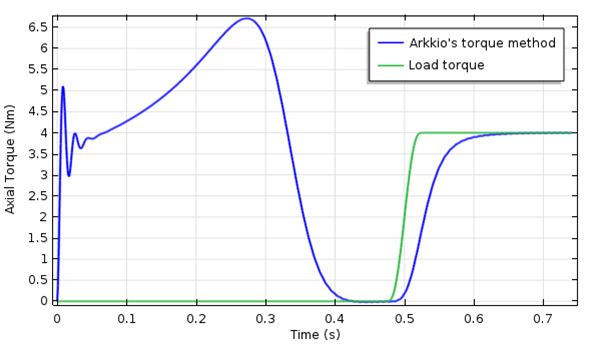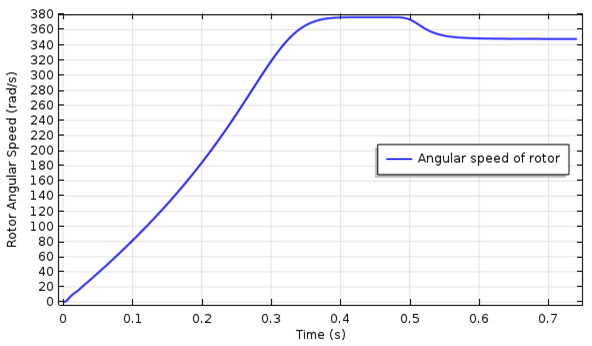### COMSOL Multiphysics 与 TEAM 问题 30a 的结果对比